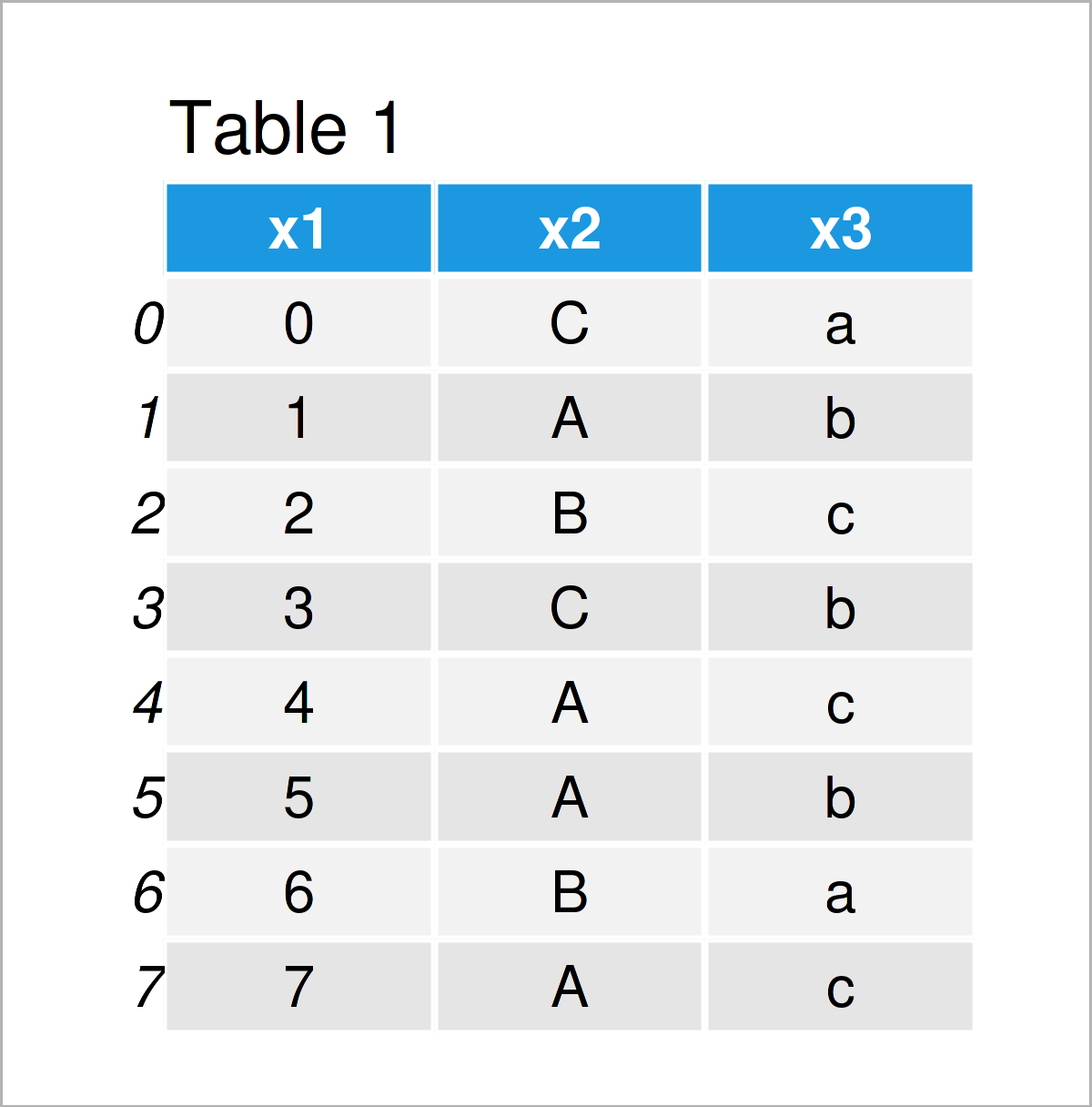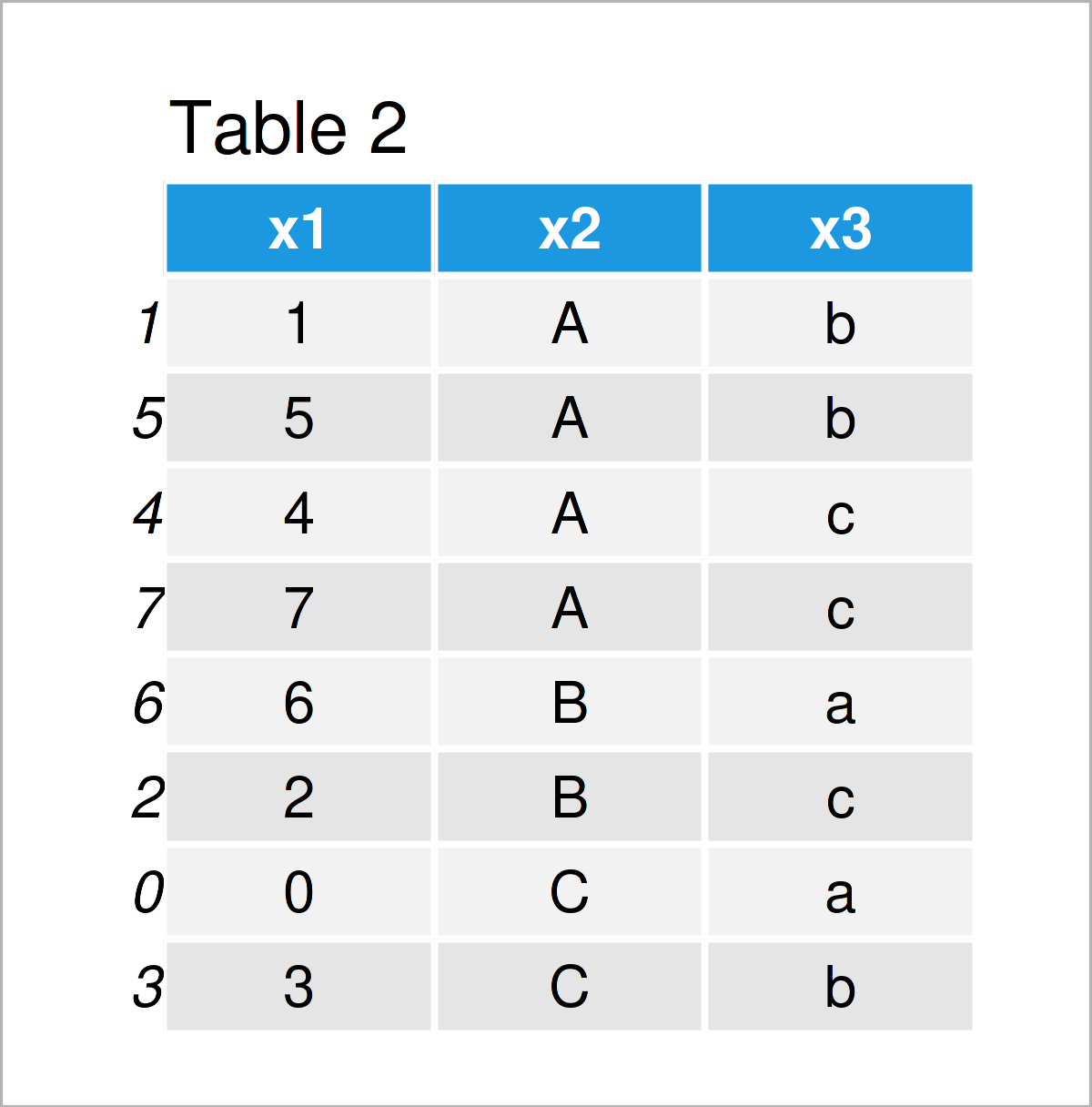# Sort pandas DataFrame by Multiple Columns in Python (Example)

This tutorial shows how to order the rows of a pandas DataFrame based on multiple columns in the Python programming language.

Sound good? Let’s get started:

## Example Data & Add-On Libraries

First, we have to import the pandas library:

`import pandas as pd # Import pandas library in Python`

We also need to create some data that we can use in the examples below:

```data = pd.DataFrame({'x1':range(0, 8), # Create example DataFrame 'x2':['C', 'A', 'B', 'C', 'A', 'A', 'B', 'A'], 'x3':['a', 'b', 'c', 'b', 'c', 'b', 'a', 'c']}) print(data) # Print example DataFrame```Table 1 shows that our exemplifying data is constituted of eight rows and three columns.

The variables x2 and x3 contain characters that we will use in the following example to rearrange the rows of our data set.

## Example: Ordering pandas DataFrame Based On Multiple Columns Using sort_values() Function

In this example, I’ll show how to sort the rows of a pandas DataFrame by two or more columns.

For this, we can use the sort_values function. Within the sort_values function, we have to specify the column names based on which we want to sort our data as shown below:

```data_new = data.sort_values(['x2', 'x3']) # Sort DataFrame print(data_new) # Print new DataFrame```Table 2 shows the output of the previously shown Python programming syntax – A new pandas DataFrame where the rows have been sorted according to the columns x2 and x3.

## Video, Further Resources & Summary

Do you need further explanations on the contents of this article? Then I recommend watching the following video on the YouTube channel of Corey Schafer. He’s explaining how to sort pandas DataFrames in Python based on different examples as in this tutorial.

Please accept YouTube cookies to play this video. By accepting you will be accessing content from YouTube, a service provided by an external third party.If you accept this notice, your choice will be saved and the page will refresh.

Furthermore, you may have a look at some of the other tutorials on this homepage. I have published numerous tutorials already.

To summarize: You have learned in this tutorial how to sort the rows of a pandas DataFrame based on multiple variables in the Python programming language. Please tell me about it in the comments section below, if you have additional comments or questions.

Subscribe to the Statistics Globe Newsletter